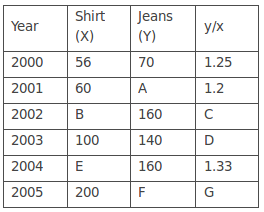## Data Interpretation For SBI PO : Set – 34

D.1-5) The following table gives the sales of batteries manufactured by a company over the years. Number of Different Types of Batteries Sold by a Company Over the Years (Numbers in Thousands)1) What was the approximate percentage increase in the sales of 55AH batteries in 1998 compared to that in 1992?

a) 28%

b) 31%

c) 33%

d) 34%

e) none

d)

(145-108)/108*100 = 34.26% = 34%

2) The total sales of all the seven years is the maximum for which battery?

a) 4AH

b) 7AH

c) 32AH

d) 35AH

e) None

c)

The total sales (in thousands) of all the seven years for various batteries are:

For 4AH = 75 + 90 + 96 + 105 + 90 + 105 + 115 = 676

For 7AH = 144 + 126 + 114 + 90 + 75 + 60 + 85 = 694

For 32AH = 114 + 102 + 75 + 150 + 135 + 165 + 160 = 901

For 35AH = 102 + 84 + 105 + 90 + 75 + 45 + 100 = 601

For 55AH = 108 + 126 + 135 + 75 + 90 + 120 + 145 = 799.

Clearly, sales are maximum in case of 32AH batteries.

3) What is the difference in the number of 35AH batteries sold in 1993 and 1997?

a) 24000

b) 28000

c) 35000

d) 39000

e) none

d)

Required Difference =[(84 -45)*1000]=39000

4) The percentage of 4AH batteries sold to the total number of batteries sold was maximum in the year?

a) 1994

b) 1995

c) 1996

d) 1997

e) none

d)

The percentages of sales of 4AH batteries to the total sales in different years are:

For 1992 = (75 /543x 100) % = 13.81%.

For 1993 = ( 90/528 x 100) % = 17.05%.

For 1994 = (96/525 x 100 )% = 18.29%.

For 1995 = ( 105/510 x 100)% = 20.59%.

For 1996 = ( 96/465 x 100 )% = 19.35%.

For 1997 = (105/495 x 100)% = 21.21%.

For 1998 = (115/605 x 100)% = 19.01%.

Clearly, the percentage is maximum in 1997.

5) In case of which battery there was a continuous decrease in sales from 1992 to 1997 ?

a) 4AH

b) 7AH

c) 32AH

d) 35AH

e) None

b) From the table it is clear that the sales of 7AH batteries have been decreasing continuously from 1992 to 1997.

D.6-10) Study the following table carefully and answer the questions that follow. A few data are assigned alphabetically:

(Sales of shirts and jeans sold by Co. X in various years, Rs. Crores)6) The per cent increase in the shirts sale between years2002 and 2004 is:

a) 40%

b) 50%

c) 45%

d) 60%

e) Can’t say

e)

B cannot be Found from Given data

7) The per cent increase in the jeans sale between 2001 and 2005 is:

a) 75%

b) 37.5%

c) 375%

d) 750%

e) Can’t be determined

e)

F cannot be found from given data

8) If G = 1.5, what per cent is sales of jeans in 2005 of sales of jeans in 2002?

a) 150

b) 175

c) 187.5

d) 160

e) 190.5

c)

F/200 = 1.5

F = 300

Required Percentage = 187.5

9) If C : D = 1 : 2 then 7B = ?

a) 1500

b) 1600

c) 1800

d) 1230

e) 1430

C/D = 1/2

C= 0.7

160/B = 0.7

B = 1660/7

7B = 1600

10) The Value of A:E is

a) 0.1

b) 0.6

c) 0.8

d) Cannot be determined

e) None of these